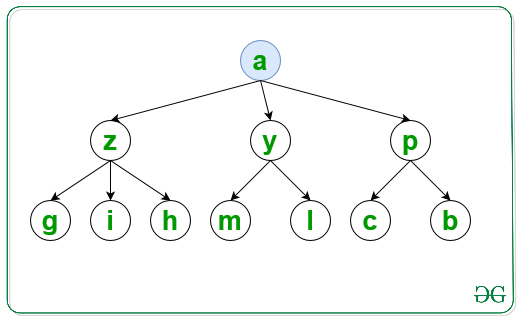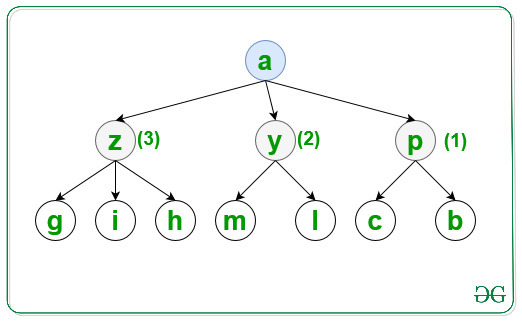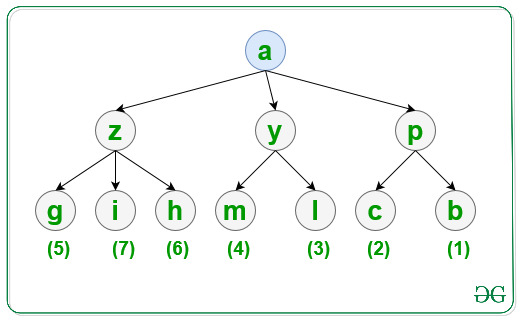# Traversal of a Graph in lexicographical order using BFS

• Last Updated : 20 Jul, 2021

Given a graph, G consisting of N nodes, a source S, and an array Edges[] of type {u, v} that denotes that there is an undirected edge between node u and v, the task is to traverse the graph in lexicographical order using BFS.

Input: N = 10, M = 10, S = ‘a’, Edges[] = { { ‘a’, ‘y’ }, { ‘a’, ‘z’ }, { ‘a’, ‘p’ }, { ‘p’, ‘c’ }, { ‘p’, ‘b’ }, { ‘y’, ‘m’ }, { ‘y’, ‘l’ }, { ‘z’, ‘h’ }, { ‘z’, ‘g’ }, { ‘z’, ‘i’ } }
Output: a p y z b c l m g h i

Explanation:
For the first level visit the node in lexicographical order as shown and print it:For the second level visit the node in lexicographical order as shown and print it:For the third level visit the node in lexicographical order as shown and print it:Input: N = 6, S = ‘a’, Edges[] = { { ‘a’, ‘e’ }, { ‘a’, ‘d’ }, { ‘e’, ‘b’ }, { ‘e’, ‘c’ }, { ‘d’, ‘f’ }, { ‘d’, ‘g’ } }
Output: a d e f g b c

Approach: Follow the steps below to solve the problem:

• Initialize a map, say G to store all the adjacent nodes of a node according to lexicographical order of the nodes.
• Initialize a map, say vis to check if a node is already traversed or not.
• Traverse the Edges[] array and store all the adjacent nodes of each node of the graph in G.
• Finally, traverse the graph using BFS and print the visited nodes of the graph.

Below is the implementation of the above approach:

## C++

 `// C++ program to implement``// the above approach` `#include ``using` `namespace` `std;` `// Function to traverse the graph in``// lexicographical order using BFS``void` `LexiBFS(map<``char``, set<``char``> >& G,``             ``char` `S, map<``char``, ``bool``>& vis)``{``    ``// Stores nodes of the graph``    ``// at each level``    ``queue<``char``> q;` `    ``// Insert nodes of first level``    ``q.push(S);` `    ``// Mark S as``    ``// visited node``    ``vis[S] = ``true``;` `    ``// Traverse all nodes of the graph``    ``while` `(!q.empty()) {` `        ``// Stores top node of queue``        ``char` `top = q.front();` `        ``// Print visited nodes of graph``        ``cout << top << ``" "``;` `        ``// Insert all adjacent nodes``        ``// of the graph into queue``        ``for` `(``auto` `i = G[top].begin();``             ``i != G[top].end(); i++) {` `            ``// If i is not visited``            ``if` `(!vis[*i]) {` `                ``// Mark i as visited node``                ``vis[*i] = ``true``;` `                ``// Insert i into queue``                ``q.push(*i);``            ``}``        ``}` `        ``// Pop top element of the queue``        ``q.pop();``    ``}``}` `// Utility Function to traverse graph``// in lexicographical order of nodes``void` `CreateGraph(``int` `N, ``int` `M, ``int` `S,``                 ``char` `Edges[])``{``    ``// Store all the adjacent nodes``    ``// of each node of a graph``    ``map<``char``, set<``char``> > G;` `    ``// Traverse Edges[] array``    ``for` `(``int` `i = 0; i < M; i++) {``        ``G[Edges[i]].insert(Edges[i]);``    ``}` `    ``// Check if a node is already visited or not``    ``map<``char``, ``bool``> vis;` `    ``LexiBFS(G, S, vis);``}` `// Driver Code``int` `main()``{``    ``int` `N = 10, M = 10 S = ``'a'``;``    ``char` `Edges[M]``        ``= { { ``'a'``, ``'y'` `}, { ``'a'``, ``'z'` `},``            ``{ ``'a'``, ``'p'` `}, { ``'p'``, ``'c'` `},``            ``{ ``'p'``, ``'b'` `}, { ``'y'``, ``'m'` `},``            ``{ ``'y'``, ``'l'` `}, { ``'z'``, ``'h'` `},``            ``{ ``'z'``, ``'g'` `}, { ``'z'``, ``'i'` `} };` `    ``// Function Call``    ``CreateGraph(N, M, S, Edges);` `    ``return` `0;``}`

## Java

 `// Java program to implement``// the above approach``import` `java.util.*;` `class` `Graph{` `// Function to traverse the graph in``// lexicographical order using BFS``static` `void` `LexiBFS(HashMap> G,``            ``char` `S, HashMap vis)``{``    ` `    ``// Stores nodes of the graph``    ``// at each level``    ``Queue q = ``new` `LinkedList<>();` `    ``// Insert nodes of first level``    ``q.add(S);` `    ``// Mark S as``    ``// visited node``    ``vis.put(S, ``true``);` `    ``// Traverse all nodes of the graph``    ``while` `(!q.isEmpty())``    ``{``        ` `        ``// Stores top node of queue``        ``char` `top = q.peek();` `        ``// Print visited nodes of graph``        ``System.out.print(top + ``" "``);` `        ``// Insert all adjacent nodes``        ``// of the graph into queue``        ``if` `(G.containsKey(top))``        ``{``            ``for``(``char`  `i : G.get(top))``            ``{``                ` `                ``// If i is not visited``                ``if` `(vis.containsKey(i))``                ``{``                    ``if` `(!vis.get(i))``                    ``{``                        ` `                        ``// Mark i as visited node``                        ``vis.put(i, ``true``);` `                        ``// Insert i into queue``                        ``q.add(i);``                    ``}``                ``}``                ``else``                ``{``                    ` `                    ``// Mark i as visited node``                    ``vis.put(i, ``true``);` `                    ``// Insert i into queue``                    ``q.add(i);``                ``}``            ``}``        ``}` `        ``// Pop top element of the queue``        ``q.remove();``    ``}``}` `// Utility Function to traverse graph``// in lexicographical order of nodes``static` `void` `CreateGraph(``int` `N, ``int` `M, ``char` `S,``                        ``char``[][] Edges)``{``    ` `    ``// Store all the adjacent nodes``    ``// of each node of a graph``    ``HashMap> G = ``new` `HashMap<>();` `    ``// Traverse Edges[] array``    ``for``(``int` `i = ``0``; i < M; i++)``    ``{``        ``if` `(G.containsKey(Edges[i][``0``]))``        ``{``            ``Set temp = G.get(Edges[i][``0``]);``            ``temp.add(Edges[i][``1``]);``            ``G.put(Edges[i][``0``], temp);``        ``}``        ``else``        ``{``            ``Set temp = ``new` `HashSet<>();``            ``temp.add(Edges[i][``1``]);``            ``G.put(Edges[i][``0``], temp);``        ``}``    ``}``    ` `    ``// Check if a node is already visited or not``    ``HashMap vis = ``new` `HashMap<>();` `    ``LexiBFS(G, S, vis);``}` `// Driver code``public` `static` `void` `main(String[] args)``{``    ``int` `N = ``10``, M = ``10``;``    ``char` `S = ``'a'``;``    ` `    ``char``[][] Edges = { { ``'a'``, ``'y'` `}, { ``'a'``, ``'z'` `},``                       ``{ ``'a'``, ``'p'` `}, { ``'p'``, ``'c'` `},``                       ``{ ``'p'``, ``'b'` `}, { ``'y'``, ``'m'` `},``                       ``{ ``'y'``, ``'l'` `}, { ``'z'``, ``'h'` `},``                       ``{ ``'z'``, ``'g'` `}, { ``'z'``, ``'i'` `} };` `    ``// Function Call``    ``CreateGraph(N, M, S, Edges);``}``}` `// This code is contributed by hritikrommie`

## Python3

 `# Python3 program to implement``# the above approach``from` `collections ``import` `deque` `G ``=` `[[] ``for` `i ``in` `range``(``1000``)]``vis ``=` `[``False` `for` `i ``in` `range``(``1000``)]` `# Function to traverse the graph in``# lexicographical order using BFS``def` `LexiBFS(S):``    ` `    ``global` `G, vis``    ` `    ``# Stores nodes of the graph``    ``# at each level``    ``q ``=` `deque()``    ` `    ``# Insert nodes of first level``    ``q.append(``ord``(S))` `    ``# Mark S as``    ``# visited node``    ``vis[``ord``(S)] ``=` `True``    ``#a = []` `    ``# Traverse all nodes of the graph``    ``while` `(``len``(q) > ``0``):``        ` `        ``# Stores top node of queue``        ``top ``=` `q.popleft()``        ``print``(``chr``(top), end ``=` `" "``)` `        ``# Insert all adjacent nodes``        ``# of the graph into queue``        ``for` `i ``in` `G[top]:``            ` `            ``# If i is not visited``            ``if` `(``not` `vis[i]):``                ``#print(chr(i),end=" ")` `                ``# Mark i as visited node``                ``vis[i] ``=` `True` `                ``# Insert i into queue``                ``q.append(i)` `# Utility Function to traverse graph``# in lexicographical order of nodes``def` `CreateGraph(N, M, S,Edges):` `    ``# Traverse Edges[] array``    ``for` `i ``in` `range``(M):``        ``G[``ord``(Edges[i][``0``])].append(``ord``(Edges[i][``1``]))` `    ``for` `i ``in` `range``(``1000``):``        ``G[i] ``=` `sorted``(G[i])` `    ``LexiBFS(S)` `# Driver Code``if` `__name__ ``=``=` `'__main__'``:``    ` `    ``N, M ``=` `10``, ``10``    ``S ``=` `'a'``    ` `    ``Edges ``=` `[ [ ``'a'``, ``'y'` `], [ ``'a'``, ``'z'` `],``              ``[ ``'a'``, ``'p'` `], [ ``'p'``, ``'c'` `],``              ``[ ``'p'``, ``'b'` `], [ ``'y'``, ``'m'` `],``              ``[ ``'y'``, ``'l'` `], [ ``'z'``, ``'h'` `],``              ``[ ``'z'``, ``'g'` `], [ ``'z'``, ``'i'` `] ]` `    ``# Function Call``    ``CreateGraph(N, M, S, Edges)` `# This code is contributed by mohit kumar 29`

## Javascript

 ``

Output:

`a p y z b c l m g h i`

Time Complexity: O(N * log(N))
Auxiliary Space: O(N)

My Personal Notes arrow_drop_up